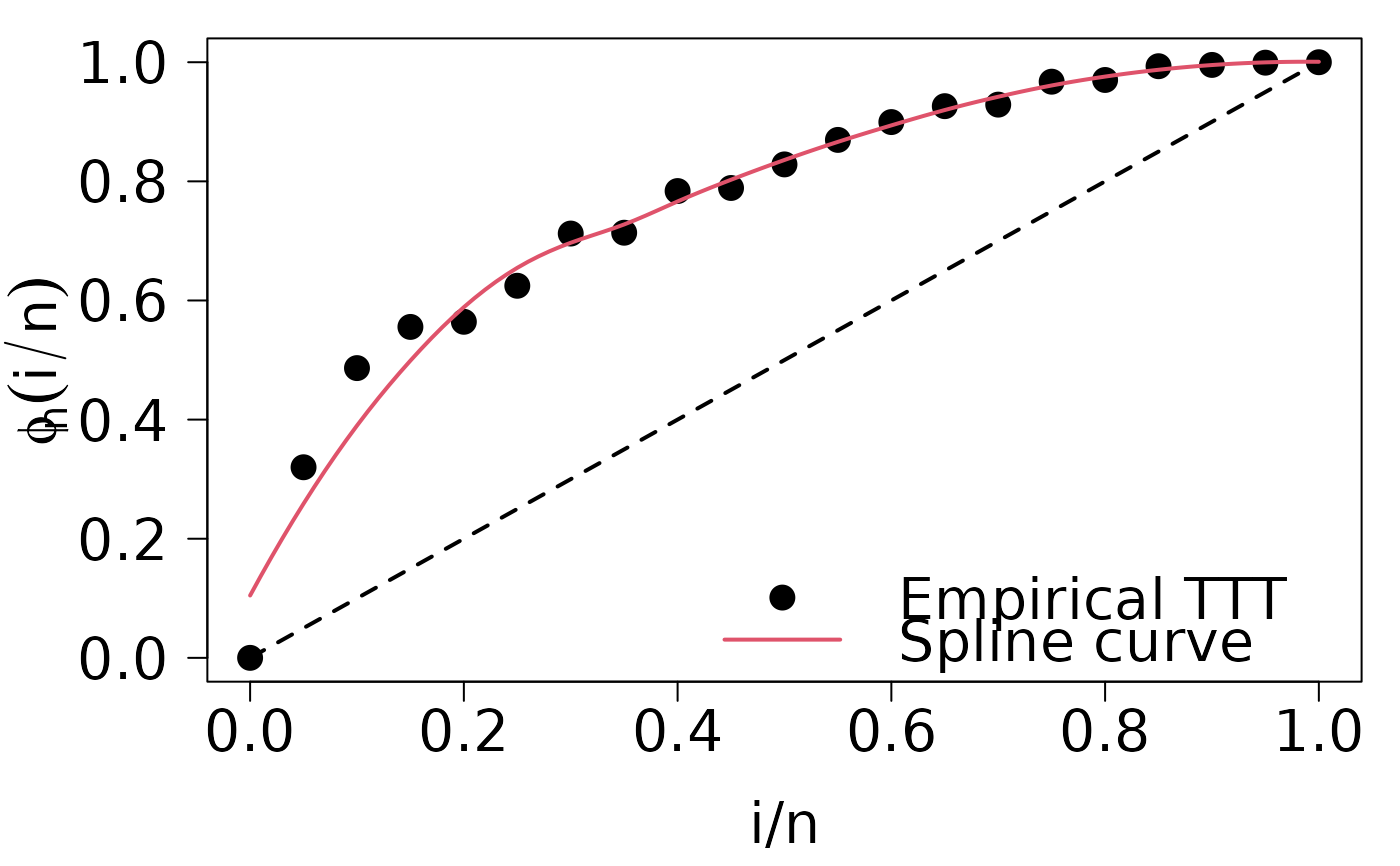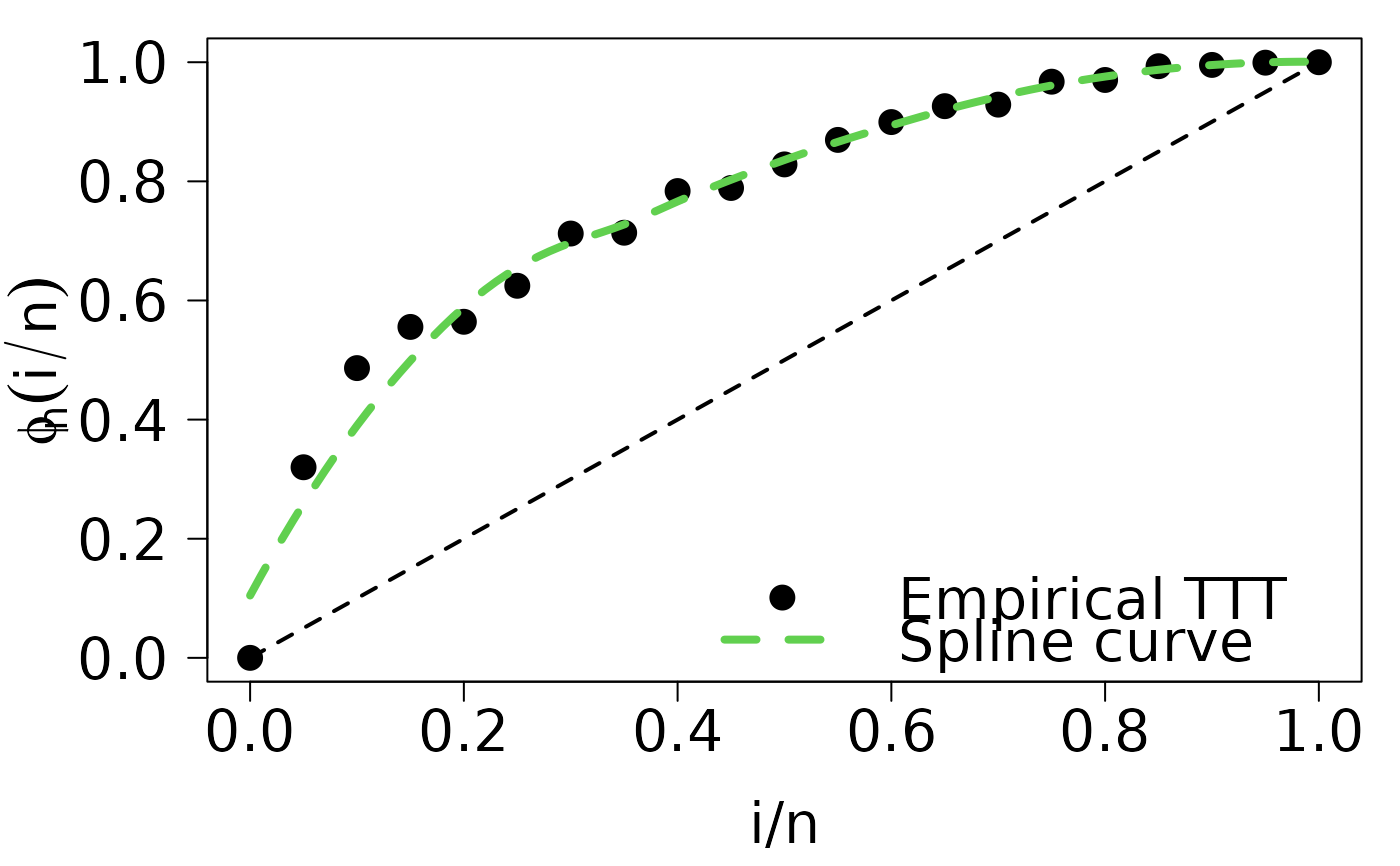Put legend after run plot.HazardShape function.

## Usage

legend.HazardShape(
x,
y = NULL,
legend = c("Empirical TTT", "Spline curve"),
fill = NULL,
col = 1,
border = "white",
lty = NA,
lwd = NA,
pch = c(1, NA),
angle = 45,
density = NULL,
bty = "o",
bg = par("bg"),
box.lwd = par("lwd"),
box.lty = par("lty"),
box.col = par("fg"),
pt.bg = NA,
cex = 1,
pt.cex = cex,
pt.lwd = lwd,
xjust = 0,
yjust = 1,
x.intersp = 1,
y.intersp = 1,
text.width = NULL,
text.col = par("col"),
text.font = NULL,
merge = TRUE,
trace = FALSE,
plot = TRUE,
ncol = 1,
horiz = FALSE,
title = NULL,
inset = 0,
xpd = TRUE,
title.col = text.col,
title.cex = cex,
title.font = text.font,
seg.len = 2,
curve_options = list(col = 2, lwd = 2, lty = 1)
)

## Arguments

x, y

the x and y co-ordinates to be used to position the legend. They can be specified by keyword or in any way which is accepted by xy.coords: See ‘Details’.

legend

a character or expression vector of length $$\ge 1$$ to appear in the legend. Other objects will be coerced by as.graphicsAnnot.

fill

if specified, this argument will cause boxes filled with the specified colors (or shaded in the specified colors) to appear beside the legend text.

col

the color of points or lines appearing in the legend.

border

the border color for the boxes (used only if fill is specified).

lty, lwd

the line types and widths for lines appearing in the legend. One of these two must be specified for line drawing.

pch

the plotting symbols appearing in the legend, as numeric vector or a vector of 1-character strings (see points). Unlike points, this can all be specified as a single multi-character string. Must be specified for symbol drawing.

angle

density

the density of shading lines, if numeric and positive. If NULL or negative or NA color filling is assumed.

bty

the type of box to be drawn around the legend. The allowed values are "o" (the default) and "n".

bg

the background color for the legend box. (Note that this is only used if bty != "n".)

box.lty, box.lwd, box.col

the line type, width and color for the legend box (if bty = "o").

pt.bg

the background color for the points, corresponding to its argument bg.

cex

character expansion factor relative to current par("cex"). Used for text, and provides the default for pt.cex.

pt.cex

expansion factor(s) for the points.

pt.lwd

line width for the points, defaults to the one for lines, or if that is not set, to par("lwd").

xjust

how the legend is to be justified relative to the legend x location. A value of 0 means left justified, 0.5 means centered and 1 means right justified.

yjust

the same as xjust for the legend y location.

x.intersp

character interspacing factor for horizontal (x) spacing between symbol and legend text.

y.intersp

vertical (y) distances (in lines of text shared above/below each legend entry). A vector with one element for each row of the legend can be used.

numeric of length 1 or 2; the string adjustment for legend text. Useful for y-adjustment when labels are plotmath expressions.

text.width

the width of the legend text in x ("user") coordinates. (Should be positive even for a reversed x axis.) Can be a single positive numeric value (same width for each column of the legend), a vector (one element for each column of the legend), NULL (default) for computing a proper maximum value of strwidth(legend)), or NA for computing a proper column wise maximum value of strwidth(legend)).

text.col

the color used for the legend text.

text.font

the font used for the legend text, see text.

merge

logical; if TRUE, merge points and lines but not filled boxes. Defaults to TRUE if there are points and lines.

trace

logical; if TRUE, shows how legend does all its magical computations.

plot

logical. If FALSE, nothing is plotted but the sizes are returned.

ncol

the number of columns in which to set the legend items (default is 1, a vertical legend).

horiz

logical; if TRUE, set the legend horizontally rather than vertically (specifying horiz overrides the ncol specification).

title

a character string or length-one expression giving a title to be placed at the top of the legend. Other objects will be coerced by as.graphicsAnnot.

inset

inset distance(s) from the margins as a fraction of the plot region when legend is placed by keyword.

xpd

if supplied, a value of the graphical parameter xpd to be used while the legend is being drawn.

title.col

color for title, defaults to text.col.

horizontal adjustment for title: see the help for par("adj").

title.cex

expansion factor(s) for the title, defaults to cex.

title.font

the font used for the legend title, defaults to text.font, see text.

seg.len

the length of lines drawn to illustrate lty and/or lwd (in units of character widths).

curve_options

a list whose names are curve graphical parameters, and whose values are the corresponding graphical parameters values.

## Details

This function is a wrapper for the legend function. It just adds two features:

• legend has a default value, regarding that HazardShape objects produces the TTT plot and its LOESS estimation.

• curve_options is used to set legend parameters for the LOESS curve. We encourage you to define first a list with curve parameters, and then pass it to plot.HazardShape and legend.HazardShape (see example 2).

## Author

Jaime Mosquera Gutiérrez jmosquerag@unal.edu.co

## Examples

library(EstimationTools)

data("reduction_cells")
TTT_IG <- TTTE_Analytical(Surv(days, status) ~ 1, data = reduction_cells,
method = 'censored')
HS_IG <- TTT_hazard_shape(TTT_IG, data = reduction_cells)

#----------------------------------------------------------------------------
# Example 1: put legend to the TTT plot of the reduction cells data set.

# Run plot.HazardShape method.
par(
cex.lab=1.8,
cex.axis=1.8,
mar=c(4.8,5.4,1,1),
las = 1,
mgp=c(3.4,1,0)
)
plot(HS_IG, pch = 16, cex = 1.8)

# Finally, put the legend
legend.HazardShape(
x = "bottomright",
box.lwd = NA,
cex = 1.8,
pt.cex = 1.8,
bty = 'n',
pch = c(16, NA)
)#----------------------------------------------------------------------------
# Example 2: example 1 with custom options for the curve

# This is the list with curve parameters
loess_options <- list(
col = 3, lwd = 4, lty = 2
)
par(
cex.lab=1.8,
cex.axis=1.8,
mar=c(4.8,5.4,1,1),
las = 1,
mgp=c(3.4,1,0)
)

plot(HS_IG, pch = 16, cex = 1.8, curve_options = loess_options)
legend.HazardShape(
x = "bottomright",
box.lwd = NA,
cex = 1.8,
pt.cex = 1.8,
bty = 'n',
pch = c(16, NA),
curve_options = loess_options
)